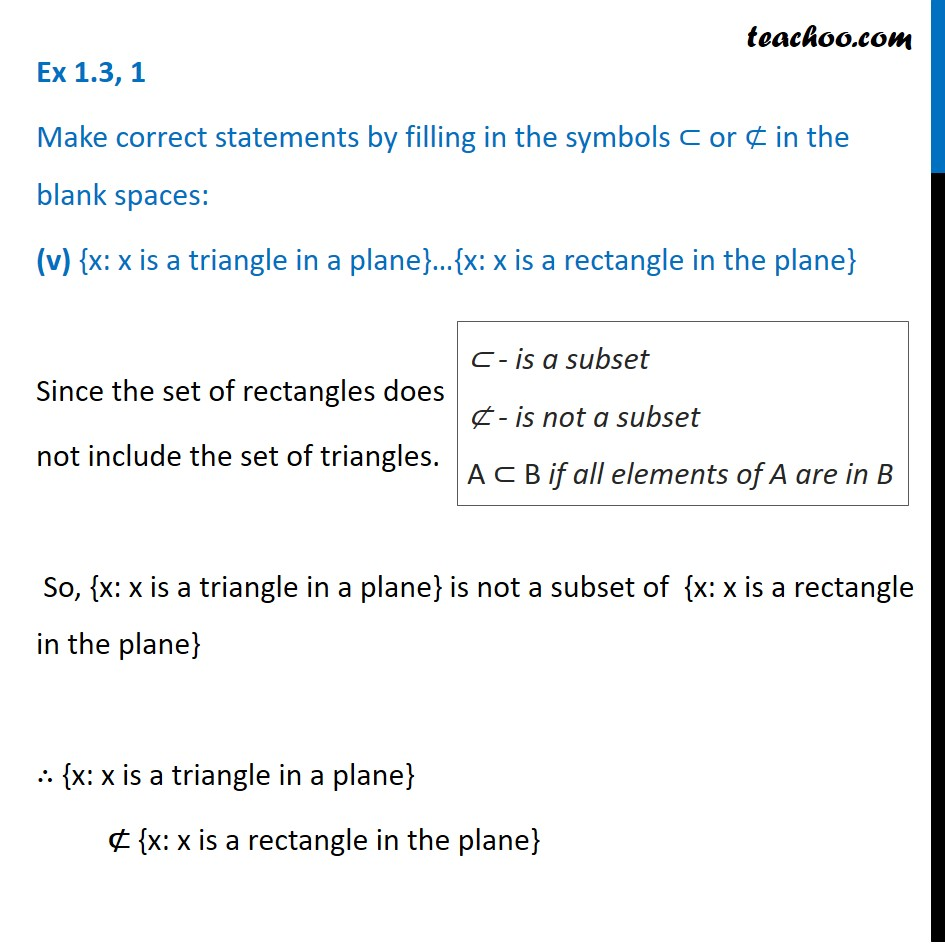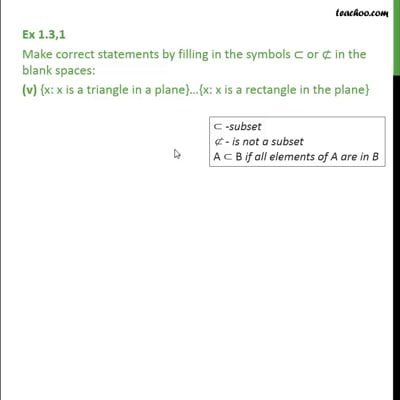Ex 1.3

Chapter 1 Class 11 Sets
Serial order wiseThis video is only available for Teachoo black users

Maths Crash Course - Live lectures + all videos + Real time Doubt solving!

### Transcript

Ex 1.3, 1 Make correct statements by filling in the symbols ⊂ or ⊄ in the blank spaces: (v) {x: x is a triangle in a plane}…{x: x is a rectangle in the plane} Since the set of rectangles does not include the set of triangles. So, {x: x is a triangle in a plane} is not a subset of {x: x is a rectangle in the plane} ∴ {x: x is a triangle in a plane} ⊄ {x: x is a rectangle in the plane} ⊂ - is a subset ⊄ - is not a subset A ⊂ B if all elements of A are in B